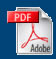Home English Maths Science ICT History Geography Art Year Six Revision Maths APP
Home > Year Six Transition

Welcome to the Year Six Transition Page.Lesson One Lesson Two Lesson Three Lesson Four Lesson FiveLesson Six Lesson Seven Lesson Eight Lesson Nine Lesson TenEratosthenes Sieve Blank Pascals Triangle Blue Pascal's Triangle Red Pascal's Triangle Gold Pascal's TriangleGreen Pascal's Triangle Pascal's Triangle Investigation Notes. Pascal's Triangle Blank (19 rows) Pascal's Triangle (19 rows Last two to fill in) Pascal's Triangle Missing numbersComplete Pascal's Triangle up to 18th row Shorter Lesson Version Shorter Lesson Version Blank